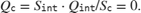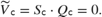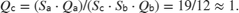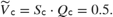## Operations for Fixed-Point Data in Stateflow

Stateflow® charts in Simulink® models have an action language property that defines the syntax that you use to compute with fixed-point data:

•MATLAB® as the action language.

•C as the action language.

For more information, see Differences Between MATLAB and C as Action Language Syntax.

### Binary Operations

This table summarizes the interpretation of all binary operations on fixed-point operands according to their order of precedence (0 = highest, 9 = lowest). Binary operations are left associative so that, in any expression, operators with the same precedence are evaluated from left to right.

Operation

Precedence

MATLAB as the Action Language

C as the Action Language

a ^ b

0

Power. Not supported for fixed-point operands defined by using either a slope that is not an integer power of two or a nonzero bias. Exponent operand must be a constant whose value is a non-negative integer.

Power. Enable this operation by clearing the Enable C-bit operations chart property. See Enable C-bit operations.

a * b

1

Multiplication. For fixed-point operands defined by using either a slope that is not an integer power of two or a nonzero bias, specify a chart fimath object with ProductMode set to SpecifyPrecision. See Multiplication.

Multiplication. Not supported for fixed-point operands defined by using a nonzero bias. See Multiplication.

a / b

1

Division. Not supported for fixed-point operands defined by using either a slope that is not an integer power of two or a nonzero bias. See Division.

Division. Not supported for fixed-point operands defined by using a nonzero bias. See Division.

a + b

2

Addition. For fixed-point operands defined by using either a slope that is not an integer power of two or a nonzero bias, specify a chart fimath object with SumMode set to SpecifyPrecision. See Addition and Subtraction.

a - b

2

Subtraction. For fixed-point operands defined by using either a slope that is not an integer power of two or a nonzero bias, specify a chart fimath object with SumMode set to SpecifyPrecision. See Addition and Subtraction.

a > b

3

Comparison, greater than. See Relational Operations for Fixed-Point Data.

Comparison, greater than. Not supported for fixed-point operands with mismatched biases. See Relational Operations for Fixed-Point Data.

a < b

3

Comparison, less than. See Relational Operations for Fixed-Point Data.

Comparison, less than. Not supported for fixed-point operands with mismatched biases. See Relational Operations for Fixed-Point Data.

a >= b

3

Comparison, greater than or equal to. See Relational Operations for Fixed-Point Data.

Comparison, greater than or equal to. Not supported for fixed-point operands with mismatched biases. See Relational Operations for Fixed-Point Data.

a <= b

3

Comparison, less than or equal to. See Relational Operations for Fixed-Point Data.

Comparison, less than or equal to. Not supported for fixed-point operands with mismatched biases. See Relational Operations for Fixed-Point Data.

a == b

4

Comparison, equal to. See Relational Operations for Fixed-Point Data.

Comparison, equal to. Not supported for fixed-point operands with mismatched biases. See Relational Operations for Fixed-Point Data.

a ~= b

4

Comparison, not equal to. See Relational Operations for Fixed-Point Data.

Comparison, not equal to. Not supported for fixed-point operands with mismatched biases. See Relational Operations for Fixed-Point Data.

a != b

4

Not supported. Use the operation a ~= b. See Relational Operations for Fixed-Point Data.

Comparison, not equal to. Not supported for fixed-point operands with mismatched biases. See Relational Operations for Fixed-Point Data.

a <> b

4

Not supported. Use the operation a ~= b. See Relational Operations for Fixed-Point Data.

Comparison, not equal to. Not supported for fixed-point operands with mismatched biases. See Relational Operations for Fixed-Point Data.

a && b

8

Logical AND. See Logical Operations for Fixed-Point Data.

Logical AND. See Logical Operations for Fixed-Point Data.

a || b

9

Logical OR. See Logical Operations for Fixed-Point Data.

Logical OR. See Logical Operations for Fixed-Point Data.

### Unary Operations and Actions

This table summarizes the interpretation of all unary operations and actions on fixed-point operands. Unary operations:

• Have higher precedence than binary operators.

• Are right associative so that, in any expression, they are evaluated from right to left.

Operation

MATLAB as the Action Language

C as the Action Language

~a

Not supported. Use the expression a == cast(0,"like",a). See Logical Operations for Fixed-Point Data.

Logical NOT. Enable this operation by clearing the Enable C-bit operations chart property. See Logical Operations for Fixed-Point Data and Enable C-bit operations.

!a

Not supported. Use the expression a == cast(0,"like",a). See Logical Operations for Fixed-Point Data.

Logical NOT. See Logical Operations for Fixed-Point Data.

-a

Negative. See Unary Minus.

Negative. See Unary Minus.

a++

Not supported. Use the expression a = a+1.

Increment. Equivalent to a = a+1.

a--

Not supported. Use the expression a = a-1.

Decrement. Equivalent to a = a-1.

### Assignment Operations

This table summarizes the interpretation of assignment operations on fixed-point operands.

Operation

MATLAB as the Action Language

C as the Action Language

a = b

Simple assignment.

Simple assignment.

a := b

Not supported. To override fixed-point promotion rules, use explicit type cast operations. See Type Cast Operations.

Special assignment that overrides fixed-point promotion rules. See Override Fixed-Point Promotion in C Charts.

a += b

Not supported. Use the expression a = a+b.

Equivalent to a = a+b.

a -= b

Not supported. Use the expression a = a-b.

Equivalent to a = a-b.

a *= b

Not supported. Use the expression a = a*b.

Equivalent to a = a*b.

a /= b

Not supported. Use the expression a = a/b.

Equivalent to a = a/b.

#### Override Fixed-Point Promotion in C Charts

In charts that use C as the action language, a simple assignment of the form a = b calculates an intermediate value for b according to the fixed-point promotion rules. Then this intermediate value is cast to the type of a by using an online conversion. See Promotion Rules for Fixed-Point Operations and Conversion Operations. Simple assignments are most efficient when both types have equal bias and slopes that either are equal or are both powers of two.

In contrast, a special assignment of the form a := b overrides this behavior by initially using the type of a as the result type for the value of b.

• Constants in b are converted to the type of a by using offline conversions.

• The expression b can contain at most one arithmetic operator (+, -, *, or /). The result is determined by using an online conversion.

• If b contains anything other than an arithmetic operation or a constant, then the special assignment operation behaves like the simple assignment operation (=).

Use the special assignment operation := when you want to:

Note

Using the special assignment operation := can result in generated code that is less efficient than the code you generate by using the normal fixed-point promotion rules.

#### Avoid Overflow in Fixed-Point Addition

You can use the special assignment operation := to avoid overflow when performing an arithmetic operation on two fixed-point numbers. For example, consider a chart that computes the sum a+b where a = 212-1 = 4095 and b = 1.

Suppose that:

• Both inputs are signed 16-bit fixed-point numbers with three fraction bits (type fixdt(1,16,3)).

• The output c is a signed 32-bit fixed-point number with three fraction bits (type fixdt(1,32,3)).

• The integer word size for production targets is 16 bits.

Because the target integer size is 16 bits, the simple assignment c = a+b adds the inputs in 16 bits before casting the sum to 32 bits. The intermediate result is 4096, which, as a type fixdt(1,16,3) value, results in an overflow.

In contrast, the special assignment c := a+b casts the inputs to 32 bits before computing the sum. The result of 4096 is safely computed as a type fixdt(1,32,3) value without an overflow.

#### Improve Precision in Fixed-Point Division

You can use the special assignment operation := to obtain a more precise result when multiplying or dividing two fixed-point numbers. For example, consider a chart that computes the ratio a/b where a = 2 and b = 3.

Suppose that:

• The input a is a fixed-point number with four fraction bits (type fixdt(1,16,4)).

• The input b is a fixed-point number with three fraction bits (type fixdt(1,16,3)).

• The output c is a signed 16-bit fixed-point number with six fraction bits (type fixdt(1,16,6)).

The inputs correspond to these slopes and quantized integers:

Sa = 2–4, Qa = 32

Sb = 2–3, Qb = 24.

The simple assignment c = a/b first calculates an intermediate value for a/b according to the fixed-point promotion rules. The quantized integer is rounded to the floor:

Sint = Sa/Sb = 2-4/2-3 = 2-1

Qint = Qa/Qb = 32/24 ≈ 1.

The intermediate result is then cast as a signed 16-digit fixed-point number with six fraction bits:

Sc = 2-6 = 1/64

Qc = SintQint/Sc = 2-1/2-6 = 25 = 32.

Therefore, the approximate real-world value for c is VcScQc = 32/64 = 0.5. This result is not a good approximation of the actual value of 2/3.

In contrast, the special assignment c := a/b calculates a/b directly as a signed 16-digit fixed-point number with six fraction bits. Again, the quantized integer is rounded to the floor:

Sc = 2-6 = 1/64

Qc = (SaQa)/(ScSbQb) = 128/3 ≈ 42.

Therefore, the approximate real-world value for c is VcScQc = 42/64 = 0.6563. This result is a better approximation to the actual value of 2/3.

### Compare Results of Fixed-Point Arithmetic

This example shows the difference between various implementations of fixed-point arithmetic in Stateflow charts. The model contains three charts that calculate the ratio a/b where a = 19 and b = 24. Both inputs are signed 16-digit fixed-point numbers with one fraction bit (type fixdt(1,16,1)). They correspond to these slopes and quantized integers:The model calculates the value of a/b as a floating-point number of type fixdt(1,16,1) in three different ways:

• A type casting operation in a chart that uses MATLAB as the action language.

• A simple assignment operation in a chart that uses C as the action language.

• A special assignment operation in a chart that uses C as the action language.Type Casting in Chart That Uses MATLAB as the Action Language

The chart at the top of the model computes an intermediate value for a/b. The quantized integer for the intermediate value is rounded to the nearest integer:The intermediate value is then cast as a signed 16-digit fixed-point number c with one fraction bit:The output value from this chart isSimple Assignment in Chart That Uses C as the Action Language

The middle chart also computes an intermediate value for a/b. In this case, the quantized integer for the intermediate value is rounded to the floor:The intermediate value is then cast as a signed 16-digit fixed-point number c with one fraction bit:The output value from this chart isSpecial Assignment in Chart That Uses C as the Action Language

The chart at the bottom of the model uses a special assignment of the form c := a/b. The value of the division is calculated directly as a signed 16-digit fixed-point number with one fraction bit. The quantized integer is rounded to the floor:Therefore, the output value from this chart isThe three results exhibit loss of precision compared to the floating-point answer of 19/24 = 0.7917. To minimize the loss of precision to an acceptable level in your application, adjust the encoding scheme in your fixed-point data.